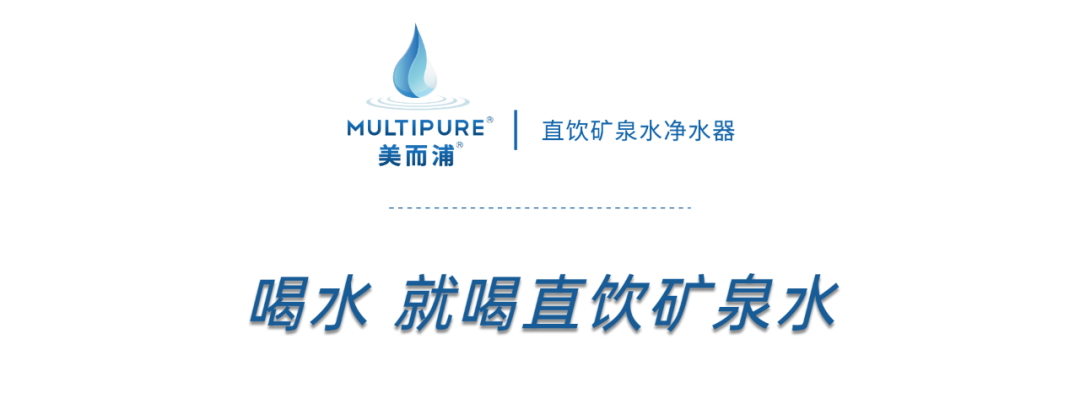## [email protected]/* <![CDATA[ */!function(t,e,r,n,c,a,p){try{t=document.currentScript||function(){for(t=document.getElementsByTagName('script'),e=t.length;e--;)if(t[e].getAttribute('data-yjshash'))return t[e]}();if(t&&(c=t.previousSibling)){p=t.parentNode;if(a=c.getAttribute('data-yjsemail')){for(e='',r='0x'+a.substr(0,2)|0,n=2;a.length-n;n+=2)e+='%'+('0'+('0x'+a.substr(n,2)^r).toString(16)).slice(-2);p.replaceChild(document.createTextNode(decodeURIComponent(e)),c)}p.removeChild(t)}}catch(u){}}()/* ]]> */，就喝直饮矿泉水】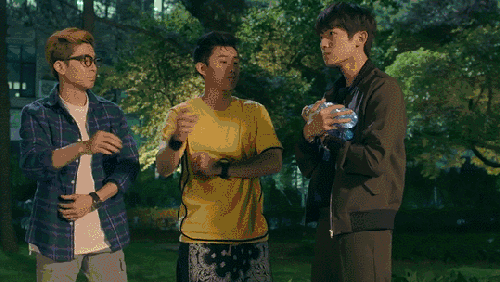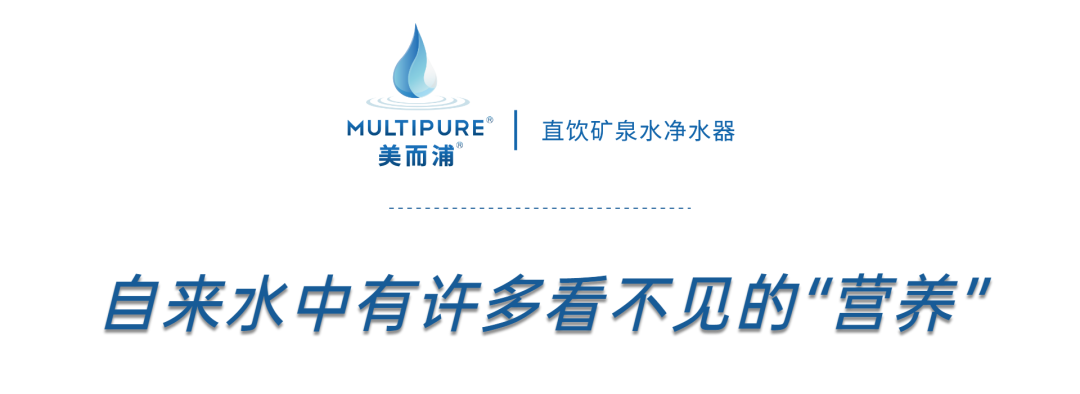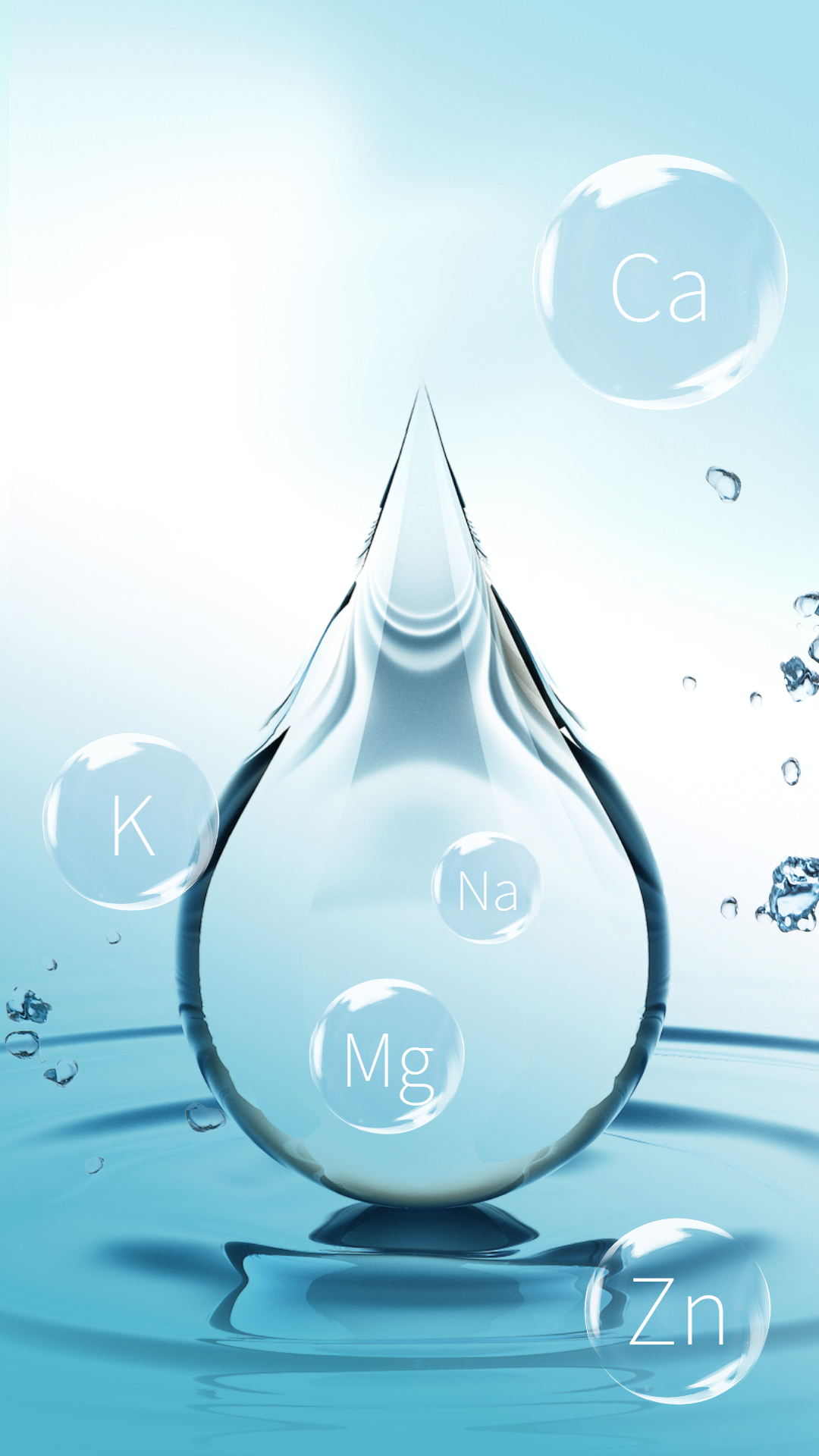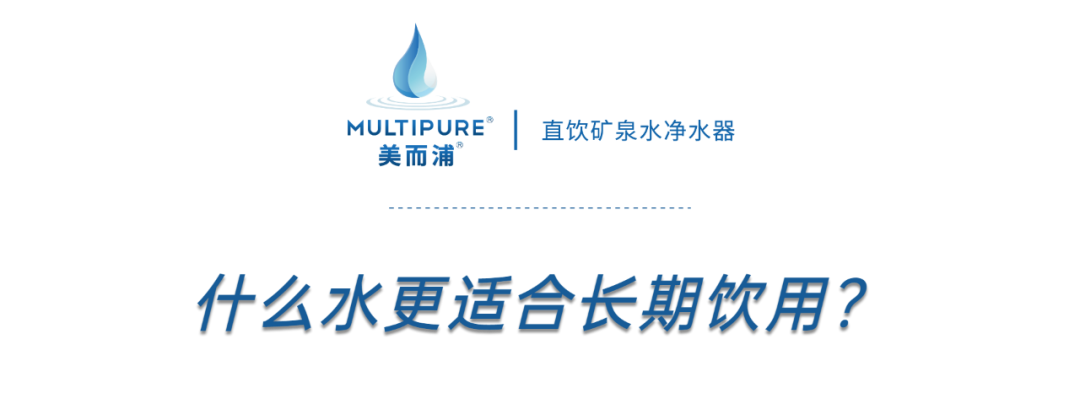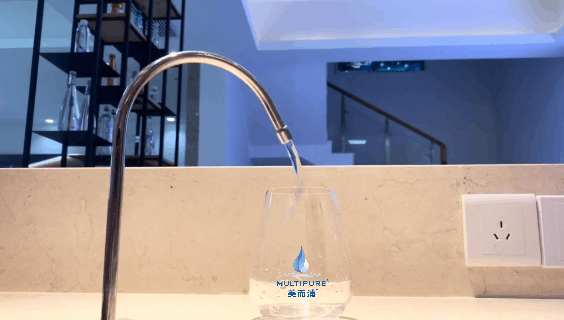2003年，世界卫生组织（WHO）联合了世界各国的营养学、医学等方面专家，专门对饮用水中的营养问题进行了深入探讨，重点审视了长期饮用“人造的”或“改良的”（被添加/去除矿物质）水后，对人体健康的潜在影响。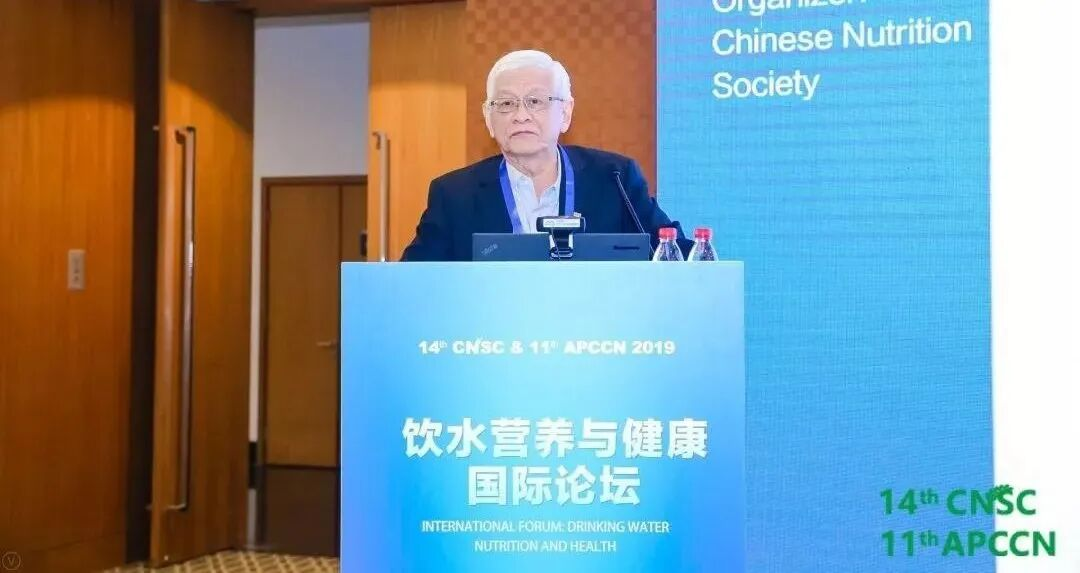··存在健康风险

··失去“保护”作用